Author: Oscar Cronquist Article last updated on August 08, 2019

Have you ever tried to build a formula to calculate discounts depending on price? The VLOOKUP function is much easier to use than nested IF functions.

You can also use the methods described in this article to calculate commissions, tariffs, charges, shipping costs, packaging expenses etc.

How do I use different price ranges to determine a discount?

For this example our price range plan looks like this:

• Total price between 0 and 100 returns a 5% discount
• Total price between 100 and 300 returns a 7% discount
• Total price between 300 and 500 returns a 8% discount
• Total price between 500 and 1000 returns a 10% discount
• Total price between 1000 and above returns a 15% discount

The picture below shows you the discount percentage table (B2:D7) and a summary table (B9:E19) with a calculated discount (E21).

The table above shows you the total amount of all items \$1 332.60 in cell E20, the formula in cell E21 calculates a discount percentage. Since \$1 332.60 is larger than \$1 000 the formula returns 15%.

Cell E23 returns the discounted total.

Keep in mind, the values in the first column (B3:B7) must be sorted ascending. The third column (D3:D7) is not necessary for the formula calculation.

Formula in cell E21:

=VLOOKUP(E20,B3:C7,2,TRUE)

Formula in cell E22:

=E20*E21

Formula in cell E23:

=E20-E22

The following chart shows you the different discount ranges from table above.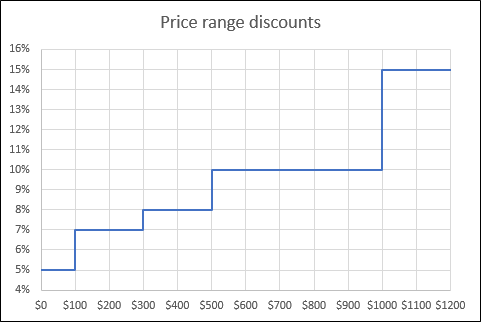How do I calculate a discount based on a linear equation?

In this scenario we want to use a linear equation to calculate the discount percentage. It begins on 0 (zero) with 2% and climbs up to 15% on \$2 000, shown in cell range B3:C4 on the picture below.

These four numbers are our two coordinates. A coordinate in a plane has two values, x and y. You can draw a line between two coordinates thus a linear equation.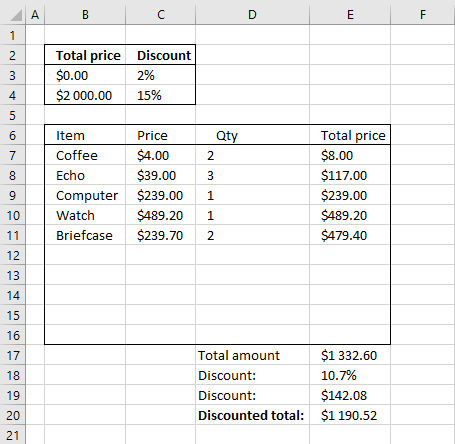Formula in cell E18:

=(C4-C3)/(B4-B3)*E17+(C3-((C4-C3)/(B4-B3))*B3)

You can verify the calculated discount value by looking at this chart.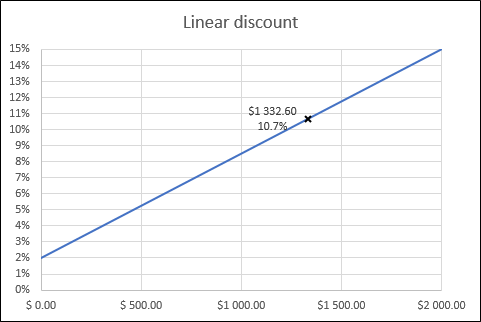What is the formula above doing?

A linear equation looks like this: y=kx+m where k and m are constants. k is the slope of the line and m is the y-coordinate where the line crosses the y-axis.

A linear equation consists of two coordinates, in this case (0,0.02) and (2000,0.15).

k is (y2-y1)/(x2-x1) or using cell refs (C4-C3)/(B4-B3), x is cell E17.

m equals y if x is zero or using cell refs C3-((C4-C3)/(B4-B3))*B3

y = kx + m becomes  (C4-C3)/(B4-B3)*E17+C3-((C4-C3)/(B4-B3))*B3

How can I use multiple tables to calculate a discount based on different price levels?

This example demonstrates how to use two different tables. The discount percentage depends on the category, the first table is category A and the second table is category B.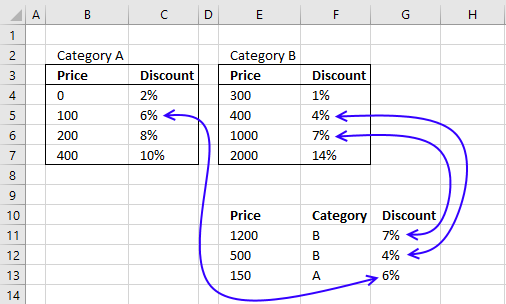Formula in cell G11:

=VLOOKUP(E11,INDEX((\$B\$4:\$C\$7,\$E\$4:\$F\$7),,,MATCH(F11,{"A","B"},0)),2,TRUE)

Explaining function in cell G11

You can easily follow the formula calculations, step by step.

1. Select cell G11
2. Go to tab "Formulas" on the ribbon
3. Click "Evaluate formula" button

Click on "Evaluate" button to see next step in calculation, click OK when done.

Step 1 - Identify relative position of category in array

MATCH(F11,{"A","B"},0)

becomes

MATCH("B",{"A","B"},0)

and returns 2. B is the second number in the array {"A","B"}. In other words, the MATCH function converts the category to a number. The number determines which range to be used.

Step 2 - Choose cell range

INDEX((\$B\$4:\$C\$7,\$E\$4:\$F\$7),,,MATCH(F11,{"A","B"},0))

becomes

INDEX((\$B\$4:\$C\$7,\$E\$4:\$F\$7),,,2)

and returns \$E\$4:\$F\$7.  The INDEX function returns cell range \$B\$4:\$C\$7 or \$E\$4:\$F\$7 depending on the category. An entire range is returned because both row and column parameters are omitted.

Step 3 - Use cell range in VLOOKUP function and return corresponding discount percentage

VLOOKUP(E11,INDEX((\$B\$4:\$C\$7,\$E\$4:\$F\$7),,,MATCH(F11,{"A","B"},0)),2,TRUE)

becomes

VLOOKUP(E11,\$E\$4:\$F\$7,2,TRUE)

becomes

VLOOKUP(1200,\$E\$4:\$F\$7,2,TRUE)

and returns 7%. The VLOOKUP function uses the selected range to retrieve the discount value from column 2 in the second table. Make sure the leftmost column in the tables are sorted descending.

Tip! This post explains how to use multiple conditions in a VLOOKUP function:
Use multiple conditions in Vlookup

How do I calculate a bonus based on multiple linear equations?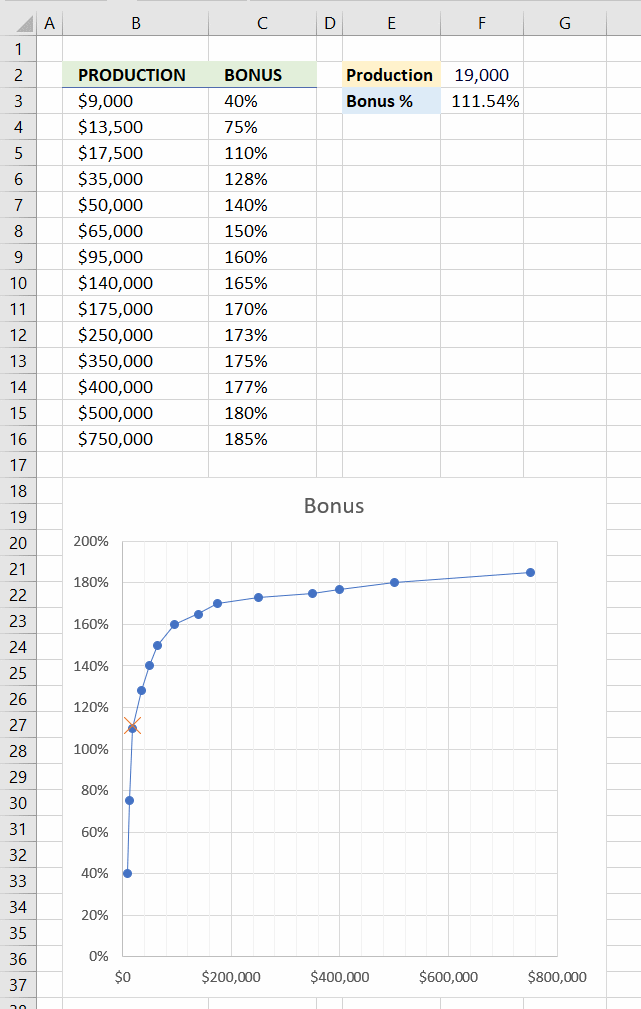This example demonstrates how to calculate a bonus based on different ranges, the bonus is linear between the levels. Please see image above.

Simply enter a value in cell F2 and if the value falls between two levels then the formula calculates the bonus based on a linear equation on those particular levels.

The value in cell F2 is 19,000 and it falls between \$17,500 and \$35,000, the bonus is 110% and 128% respectively. The number is, however, between these two numbers so the formula calculates the bonus based a line between 110% and 128%.

The chart above shows the ranges and the lines between these ranges. The VLOOKUP function can't be used in this particular example.

Formula in cell F3:

=F2*SLOPE(OFFSET(C3, MATCH(F2, B3:B16, 1)-1, , 2), OFFSET(B3, MATCH(F2, B3:B16, 1)-1, , 2))+INTERCEPT(OFFSET(C3, MATCH(F2, B3:B16, 1)-1, , 2), OFFSET(B3, MATCH(F2, B3:B16, 1)-1, , 2))

Explaining formula in cell F3

The linear equation used in this example to calculate the result is y = k*x+m

Step 1 - Identify levels based on input value and bonus table

The MATCH function allows you to get the relative position of a value in a  cell range, the table is sorted from low to high.

This allows me to find the ranges that the value falls between if I use 1 in the third argument. Please see the documentation for the MATCH function if you want to read the details.

MATCH(F2, B3:B16, 1)

becomes

MATCH(19000, {9000; 13500; 17500; 35000; 50000; 65000; 95000; 140000; 175000; 250000; 350000; 400000; 500000; 750000}, 1)

and returns 3.

Step 2 - Return values

The OFFSET function lets you return an array based on a row, column number and the size of the cell range.

OFFSET(C3, MATCH(F2, B3:B16, 1)-1, , 2)

becomes

OFFSET(C3, 3, , 2)

and returns {17500; 35000}.

Note, the OFFSET function is volatile meaning it may slow down your workbook calculations considerably if used extensively.

I could have used the INDEX function to accomplish the same task, however, the SLOPE function won't let me. The OFFSET function will do.

Step 3 - Calculate the slope

The equation we use is y = kx + m. k is the slope of the line. To calculate the slope we need two y values and two x values. k = (y2-y1)/(x2-x1)

The SLOPE function lets you calculate the slope using two or more coordinates.

SLOPE(OFFSET(C3, MATCH(F2, B3:B16, 1)-1, , 2), OFFSET(B3, MATCH(F2, B3:B16, 1)-1, , 2))

becomes

SLOPE({1.1; 1.28}, OFFSET(B3, MATCH(F2, B3:B16, 1)-1, , 2))

becomes

SLOPE({1.1; 1.28}, {17500; 35000})

and returns 0.0000102857.

Step 3 - Calculate where the line crosses the y axis

The equation we use is y = kx + m. m represents the value where the line crosses the y axis. x is 0 (zero).

The INTERCEPT function is handy in this case, it allows you to calculate the m value using two or more coordinates.

INTERCEPT(OFFSET(C3, MATCH(F2, B3:B16, 1)-1, , 2), OFFSET(B3, MATCH(F2, B3:B16, 1)-1, , 2))

becomes

INTERCEPT({1.1; 1.28}, {17500; 35000})

and returns 0.92.

Step 4 - Calculate bonus
F2*SLOPE(OFFSET(C3, MATCH(F2, B3:B16, 1)-1, , 2), OFFSET(B3, MATCH(F2, B3:B16, 1)-1, , 2))+INTERCEPT(OFFSET(C3, MATCH(F2, B3:B16, 1)-1, , 2), OFFSET(B3, MATCH(F2, B3:B16, 1)-1, , 2))

becomes

19000*0.0000102857 + 0.92

and equals 1.1154283 (111.54%).

* You will also get a weekly newsletter, unsubscribe anytime!

Two-way lookup in multiple tables

This example shows you how to do lookups in multiple tables.Formula in cell S3:

=INDEX(INDEX((\$C\$4:\$G\$8,\$J\$4:\$N\$8),,,MATCH(R3,{"North","South"},0)),MATCH(Q3,INDEX((\$B\$4:\$B\$8,\$I\$4:\$I\$8),,,MATCH(R3,{"North","South"},0)),0),MATCH(P3,INDEX((\$C\$3:\$G\$3,\$J\$3:\$N\$3),,,MATCH(R3,{"North","South"},0)),0))

Explaining formula in cell S3

The INDEX function allows you to choose between different cell ranges depending on the region value in cell R3, if a value is present in the optional parameter area_num.

You can easily follow the formula calculations, step by step.

1. Select cell S3
2. Go to tab "Formulas" on the ribbon
3. Click "Evaluate formula" button

Click on "Evaluate" button to see next step in the calculation, click OK when done.

Step 1 - Identify which cell range to fetch data from, C4:G8 or J4:N8

The MATCH function lets you find out where in an array a value is located (relative position).

INDEX((\$C\$4:\$G\$8,\$J\$4:\$N\$8),,,MATCH(R3,{"North","South"},0))

becomes

INDEX((\$C\$4:\$G\$8,\$J\$4:\$N\$8),,,1,0))

and returns the first cell reference \$C\$4:\$G\$8.

Step 2 - Identify which cell range to search in, B4:B8 or I4:I8

INDEX((\$B\$4:\$B\$8,\$I\$4:\$I\$8),,,MATCH(R3,{"North","South"},0))

becomes

INDEX((\$B\$4:\$B\$8,\$I\$4:\$I\$8),,,1)

and returns \$B\$4:\$B\$8

Step 3 - Match value "D" in cell range \$B\$4:\$B\$8

MATCH(Q3,INDEX((\$B\$4:\$B\$8,\$I\$4:\$I\$8),,,MATCH(R3,{"North","South"},0)),0)

becomes

MATCH(Q3,\$B\$4:\$B\$8,0)

becomes

MATCH("D",\$B\$4:\$B\$8,0)

and returns 4. Now we now the row number to use.

Step 4 - Find column number

The steps to look for the column number is almost identical to finding the row number.

MATCH(P3,INDEX((\$C\$3:\$G\$3,\$J\$3:\$N\$3),,,MATCH(R3,{"North","South"},0)),0)

becomes

MATCH(P3,INDEX((\$C\$3:\$G\$3,\$J\$3:\$N\$3),,,1),0)

becomes

MATCH(P3,\$C\$3:\$G\$3,0)

becomes

MATCH("G",\$C\$3:\$G\$3,0)

and returns 2.

Step 5 - Return value from intersection of a row and column number

INDEX(INDEX((\$C\$4:\$G\$8,\$J\$4:\$N\$8),,,MATCH(R3,{"North","South"},0)),MATCH(Q3,INDEX((\$B\$4:\$B\$8,\$I\$4:\$I\$8),,,MATCH(R3,{"North","South"},0)),0),MATCH(P3,INDEX((\$C\$3:\$G\$3,\$J\$3:\$N\$3),,,MATCH(R3,{"North","South"},0)),0))

becomes

INDEX(\$C\$4:\$G\$8,4,2)

and returns 63.

You can easily modify the formula to search simultaneously in 4 different cross reference tables.

Example: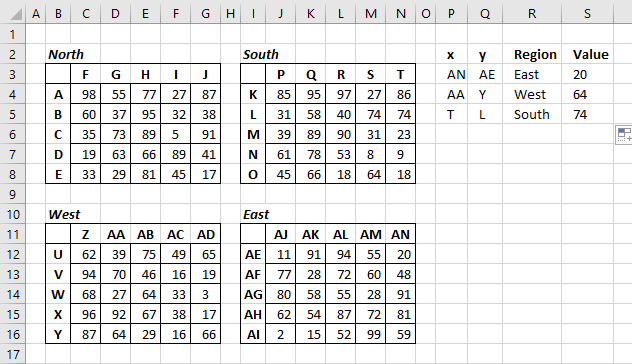Formula in cell S3:

=INDEX(INDEX((\$C\$4:\$G\$8, \$J\$4:\$N\$8, \$C\$12:\$G\$16, \$J\$12:\$N\$16), , , MATCH(R3, {"North", "South", "West", "East"}, 0)), MATCH(Q3, INDEX((\$B\$4:\$B\$8, \$I\$4:\$I\$8, \$B\$12:\$B\$16, \$I\$12:\$I\$16), , , MATCH(R3, {"North", "South", "West", "East"}, 0)), 0), MATCH(P3, INDEX((\$C\$3:\$G\$3, \$J\$3:\$N\$3, \$C\$11:\$G\$11, \$J\$11:\$N\$11), , , MATCH(R3, {"North", "South", "West", "East"}, 0)), 0))

Tip! Read this post to do Reverse two-way lookup in a cross reference table.